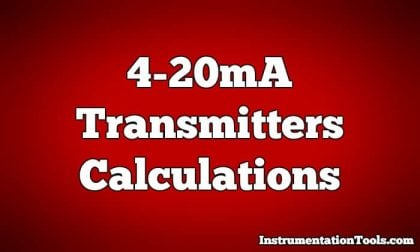Home » 4-20mA Transmitters Calculations
Formulas

4-20mA Transmitters Calculations

How-to do 4-20mA Transmitters calculations easily. This articles provides Industrial Instrumentation 4-20mA calculations with easy examples.

4-20mA Transmitters CalculationsGiven a pressure transmitter with a measurement range of 150 to 400 PSI and a signal range of 4 to 20 milliamps, calculate the applied pressure corresponding to a signal of 10.6 milliamps.

• Take 10.6 milliamps and subtract the LRV (4 milliamps),
• then divide by the span (16 milliamps) to arrive at 41.25% (0.4125 per unit).
• Take this number and multiply by the span of the pressure range (400 PSI − 150 PSI, or 250 PSI) and
• lastly add the LRV of the pressure range (150 PSI) to arrive at a ﬁnal answer of 253.125 PSI.

Given a temperature transmitter with a measurement range of −88 degrees to +145 degrees and a signal range of 4 to 20 milliamps, calculate the proper signal output at an applied temperature of +41 degrees.

• Take 41 degrees and subtract the LRV (−88 degrees) which is the same as adding 88 to 41,
• then divide by the span (145 degrees − (−88) degrees, or 233 degrees) to arrive at 55.36% (0.5536 per unit).
• Take this number and multiply by the span of the current signal range (16 milliamps) and
• lastly add the LRV of the current signal range (4 milliamps) to arrive at a ﬁnal answer of 12.86 milliamps.

Given a pH transmitter with a measurement range of 3 pH to 11 pH and a signal range of 4 to 20 milliamps, calculate the proper signal output at 9.32 pH.

• Take 9.32 pH and subtract the LRV (3 pH),
• then divide by the span (11 pH − 3 pH, or 8 pH) to arrive at 79% (0.79 per unit).
• Take this number and multiply by the span of the current signal range (16 milliamps) and
• lastly add the LRV of the current signal range (4 milliamps) to arrive at a ﬁnal answer of 16.64 milliamps.

Articles You May Like :

4-20mA Graphical Calculations

How to do Conversions Easily

Scaling Sensor Output

Level Transmitter Zero Elevation

4-20 mA from Process Variable

Transmitter Turndown Ratio, Set Span and Zero SpanOctober 16, 2017 at 5:19 am

Em đang cần tài liệu chi tiết sửa chửa hệ thống valve solenoi symoi . Mong add giúp em với.

Translated : I need detailed documentation repair solenoid valve system. Please.January 6, 2018 at 8:16 am

Dear Sir..
I want know your university name and address. From where. you did your study.
Please sir guide meApril 16, 2019 at 6:50 am

We have temp controller range 0 to 100 deg. C and it”s re-transmitting output is 4-20 ma I want to scaling PLC program y=ax+b here a is multiple factor and b is offset. Request you to please suggest value of a and b .

This website uses cookies to improve your experience. We'll assume you're ok with this, but you can opt-out if you wish. Accept Read More

WordPress Image Lightbox

Send this to a friend